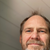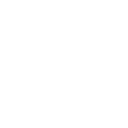0

# Cout as digital Out

christophe 5 years ago in IQANdesign updated by Ulrik Zakariasson (Software development) 5 years ago

Hi,
I want to use an Current Out channel as Digital Out ( run out of channels )
Wich function can i use to convert the on/off signal to on= current @ 100% and 0=current @ 0%?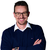Use a dual channel: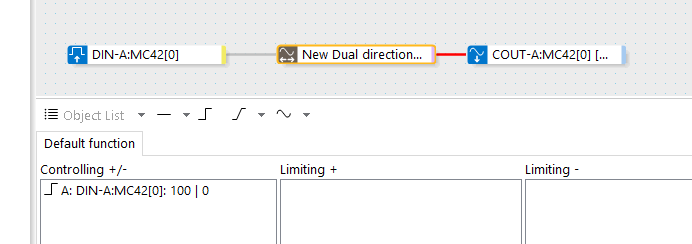You can use also both solenoids, but not at the same time. You need some interlocking, here is one way to do that:Use a dual channel:You can use also both solenoids, but not at the same time. You need some interlocking, here is one way to do that: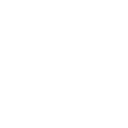Hi,

Maybe you can juste use a Math function, with the followin Q-Code :

if DIN-A = true then

Result := 100

elseif DIN-B = true then

Result := -100

endif

Or if you use just one Digital Out :

if DIN-A = true then

Result := 100

elseif DIN-A = false then

Result := -100

endif